Finding square root by division method - Integers

Chapter 5 Class 8 Squares and Square Roots
Concept wise

Let’s do this by example

### Square root of 529 by long division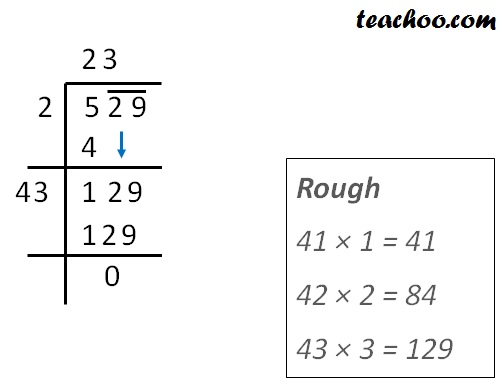Therefore,

Square root of 529 = 23

### Individual Steps are explained

Step 1:

• Write the number
• We make pairs from right.

So, 29 is a pair, but 5 is not in pair.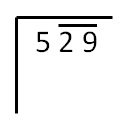Step 2:

Now we find that number whose

square is less than or equal to 5.

1 × 1 = 1

2 × 2 = 4

3 × 3 = 9

Since 9 > 5, we use 2 × 2.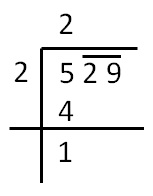So writing 2 on left side & top.

& 4 below 5

Now we find remainder,

5 − 4 = 1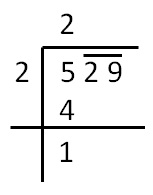Step 3

Pair 29 comes down.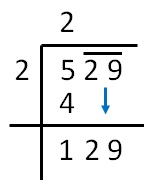Step 4

We multiply the number above by 2

& add a blank after it

So, we write 2 × 2 = 4

4 _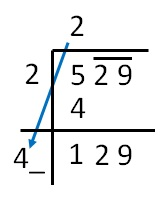Step 5

Find a number which is less than

Or equal to 129

41 × 1 = 41

42 × 2 = 84

43 × 3 = 129

Since 43 × 3 = 129. We use that

Thus,

Square root of 529 = 23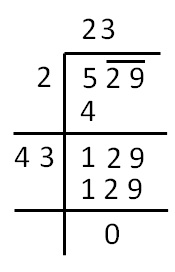### Square root of 4096 by long division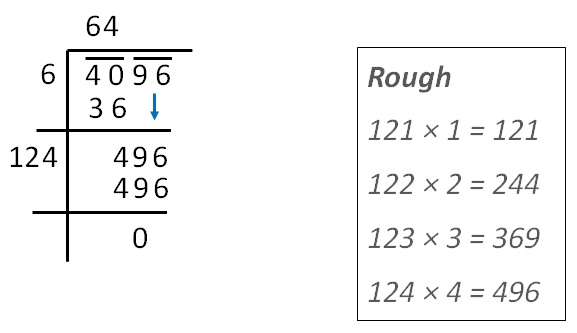Therefore,

Square root of 4096 is 64

### Individual Steps are explained

Step 1:

• Write the number
• We make pairs from right.

So, 96 and 40 are two pairs.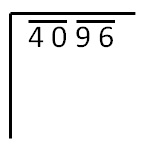Step 2:

Now, we find that number whose

square is less than or equal to 40.

5 × 5 = 25

6 × 6 = 36

7 × 7 = 49

Since 49 > 40, we use 6 × 6 = 36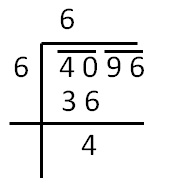So writing 6 on top and left side

& 36 below 40

Now we find remainder,

40 − 36 = 4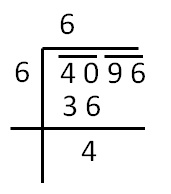Step 3

Pair 96 comes down.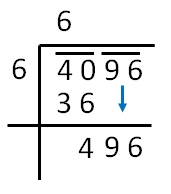Step 4

We multiply the number above

by 2 & add a blank after it

So, we write 6 × 2 = 12

12 _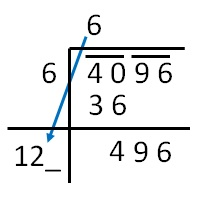Step 5

Find a number which is less than

Or equal to 496

124 × 1 = 124

124 × 2 = 248

124 × 3 = 372

124 × 4 = 496

Since 124 × 4 = 496. We use that

Thus, square root of 4096 = 64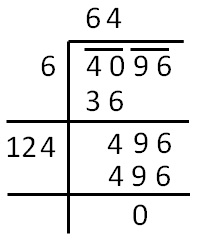Learn in your speed, with individual attention - Teachoo Maths 1-on-1 Class